# 前言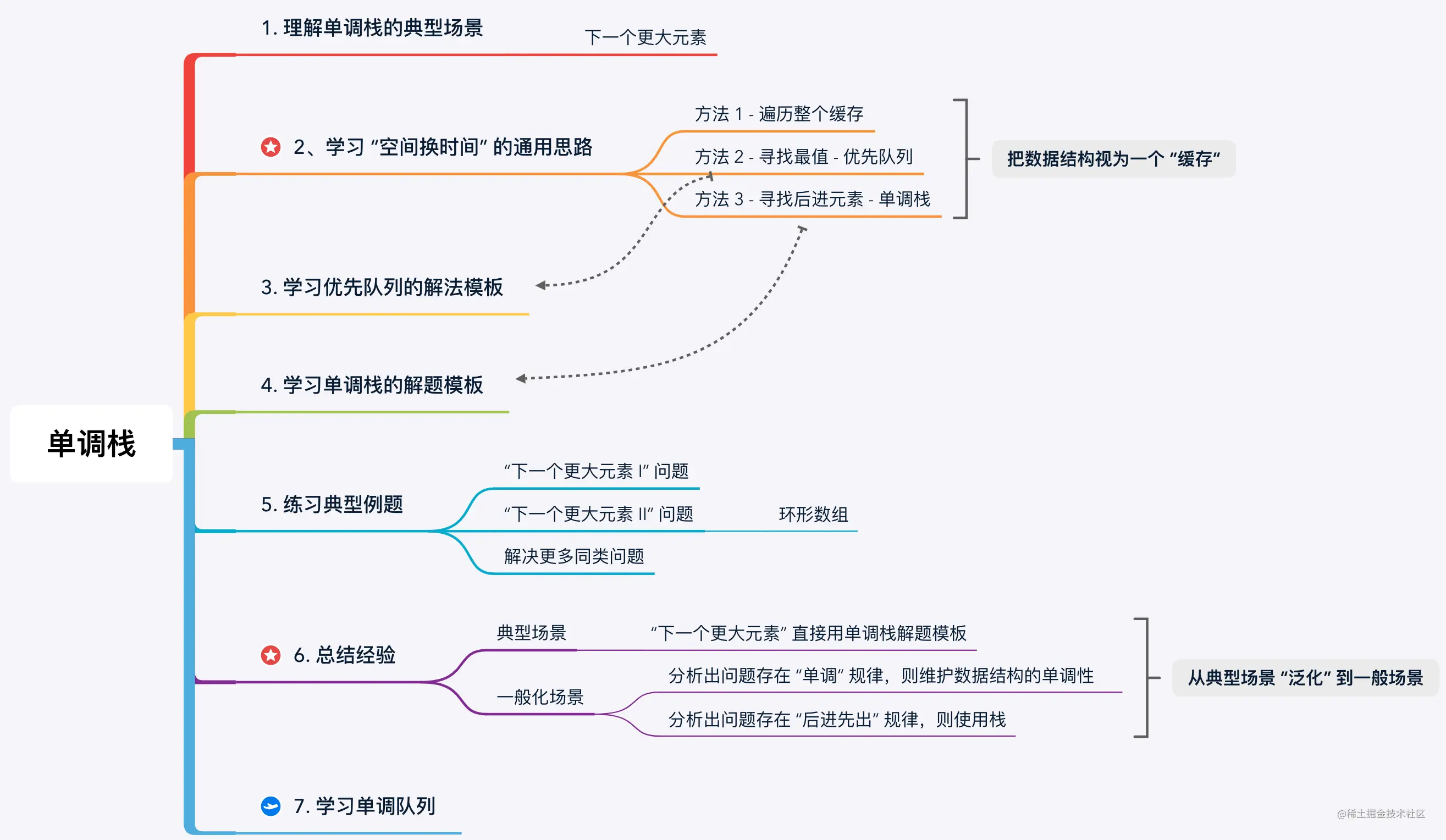# 1. 单调栈的典型问题

`形象化思考`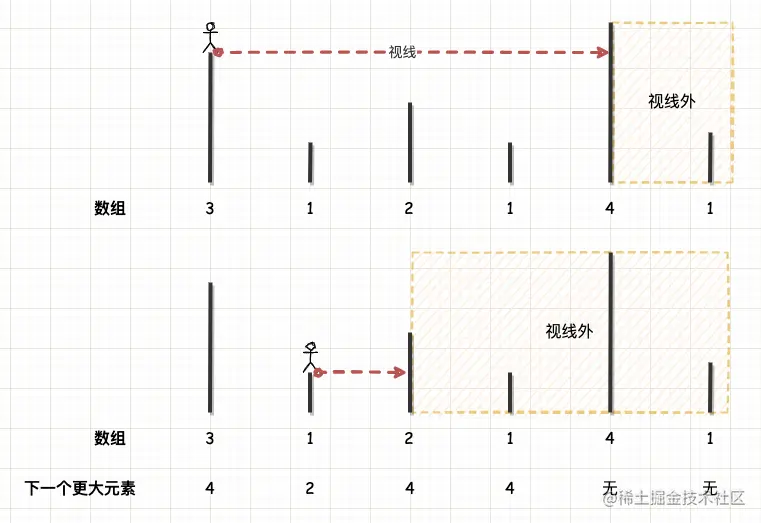# 2. 解题思路

• 在处理元素 `` 时，由于不清楚它的解，只能先将 `` 放到缓存中，继续处理下一个元素；
• 在处理元素 `` 时，我们观察缓存发现它比缓存中所有元素都小，只能先将它放到缓存中，继续处理下一个元素；
• 在处理元素 `` 时，我们观察缓存中的 `` 比当前元素小，说明当前元素就是 `` 的解。此时我们可以把 `` 从缓存中弹出，记录结果。再将 `` 放到缓存中，继续处理下一个元素；
• 在处理元素 `` 时，我们观察缓存发现它比缓存中所有元素都小，只能先将它放到缓存中，继续处理下一个元素；
• 在处理元素 `` 时，我们观察缓存中的 `` `` `` 都比当前元素小，说明当前元素就是它们的解。此时我们可以把它们从缓存中弹出，记录结果。再将 `` 放到缓存中，继续处理下一个元素；
• 在处理元素 `` 时，我们观察缓存发现它比缓存中所有元素都小，只能先将它放到缓存中，继续处理下一个元素；
• 遍历结束，从缓存中弹出过的元素都是有解的，保留在缓存中的元素都是无解的。

• 方法 1 - 暴力： 遍历整个缓存中所有元素，最坏情况（递减序列）下所有数据都进入缓存中，单次操作的时间复杂度是 O(N)O(N)O(N)，整体时间复杂度是 O(N2)O(N^2)O(N2)
• 方法 2 - 二叉堆： 不需要遍历整个缓存，只需要对比缓存的最小值，直到缓存的最小值都大于当前元素。最坏情况（递减序列）下所有数据都进入堆中，单次操作的时间复杂度是 O(lgN)O(lgN)O(lgN)，整体时间复杂度是 O(N⋅lgN)O(N·lgN)O(NlgN)
• 方法 3 - 单调栈： 我们发现元素进入缓存的顺序正好是有序的，且后进入缓存的元素会先弹出做对比，符合 “后进先出” 逻辑，所以这个缓存数据结构用栈就可以实现。因为每个元素最多只会入栈和出栈一次，所以整体的计算规模还是与数据规模成正比的，整体时间复杂度是 O(n)O(n)O(n)

# 3. 优先队列解法

• 如果堆顶元素小于当前元素，则说明已经确定了堆顶元素的解，我们将其弹出并记录结果；
• 如果堆顶元素不小于当前元素，则说明小顶堆内所有元素都是不小于当前元素的，停止观察。

`题解`

``````fun nextGreaterElements(nums: IntArray): IntArray {
// 结果数组
val result = IntArray(nums.size) { -1 }
// 小顶堆
val heap = PriorityQueue<Int> { first, second ->
nums[first] - nums[second]
}
// 从前往后查询
for (index in 0 until nums.size) {
// while：当前元素比堆顶元素大，说明找到下一个更大元素
while (!heap.isEmpty() && nums[index] > nums[heap.peek()]) {
result[heap.poll()] = nums[index]
}
// 当前元素入堆
heap.offer(index)
}
return result
}``````

• 时间复杂度： 最坏情况下（递减序列），所有元素都被添加到优先队列里，优先队列的单次操作时间复杂度是 O(lgN)O(lgN)O(lgN)，所以整体时间复杂度是 O(N⋅lgN)O(N·lgN)O(NlgN)
• 空间复杂度： 使用了额外的优先队列，所以整体的空间复杂度是 O(N)O(N)O(N)

# 4. 单调栈解法

`形象化思考`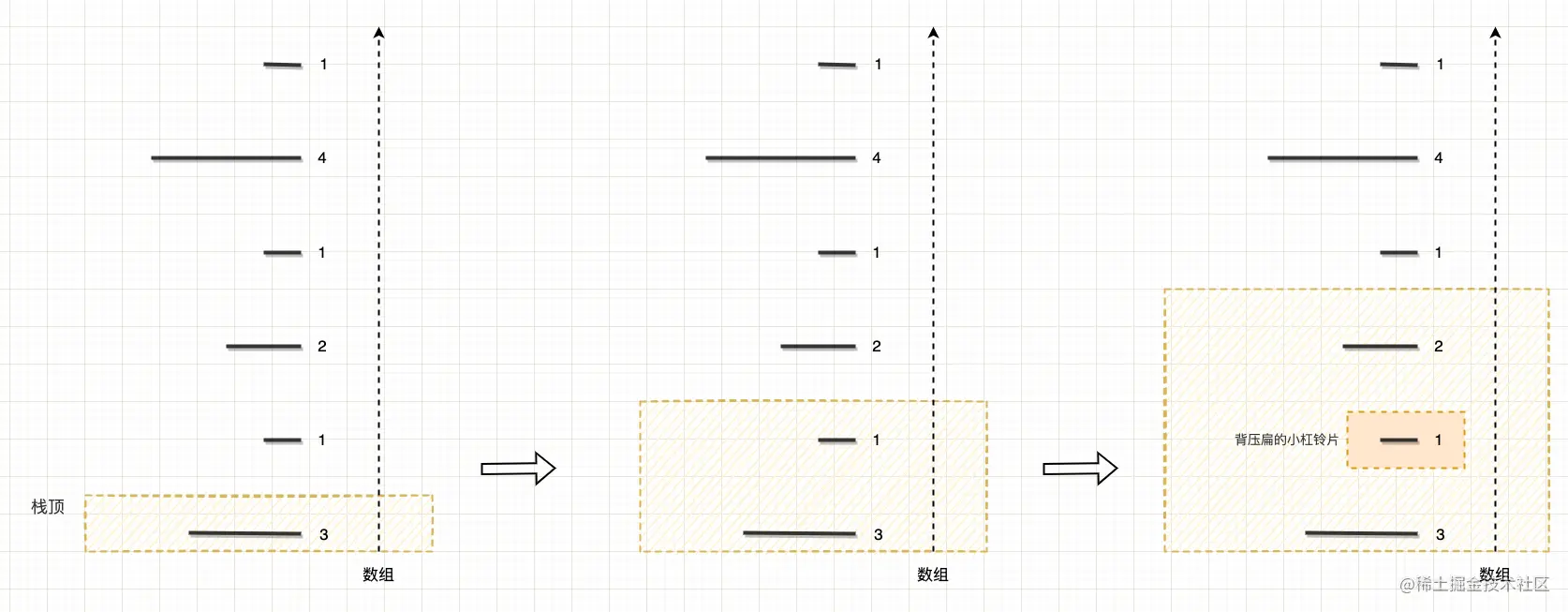`解题模板`

``````// 从前往后遍历
fun nextGreaterElements(nums: IntArray): IntArray {
// 结果数组
val result = IntArray(nums.size) { -1 }
// 单调栈
val stack = ArrayDeque<Int>()
// 从前往后遍历
for (index in 0 until nums.size) {
// while：当前元素比栈顶元素大，说明找到下一个更大元素
while (!stack.isEmpty() && nums[index] > nums[stack.peek()]) {
result[stack.pop()] = nums[index]
}
// 当前元素入队
stack.push(index)
}
return result
}``````

• 时间复杂度： 虽然代码中有嵌套循环，但它的时间复杂度并不是 O(N2)O(N^2)O(N2)，而是 O(N)O(N)O(N)。因为每个元素最多只会入栈和出栈一次，所以整体的计算规模还是与数据规模成正比的，整体时间复杂度是 O(N)O(N)O(N)
• 空间复杂度： 最坏情况下（递减序列）所有元素被添加到栈中，所以空间复杂度是 O(N)O(N)O(N)

`解题模板（从后往前遍历）`

``````// 从后往前遍历
fun nextGreaterElement(nums: IntArray): IntArray {
// 结果数组
val result = IntArray(nums.size) { -1 }
// 单调栈
val stack = ArrayDeque<Int>()
// 从后往前查询
for (index in nums.size - 1 downTo 0) {
// while：栈顶元素比当前元素小，说明栈顶元素不再是下一个更大元素，后续不再考虑它
while (!stack.isEmpty() && stack.peek() <= nums[index]) {
stack.pop()
}
// 输出到结果数组
result[index] = stack.peek() ?: -1
// 当前元素入队
stack.push(nums[index])
}
return result
}``````

# 5. 典型例题 · 下一个更大元素 I

`LeetCode 例题`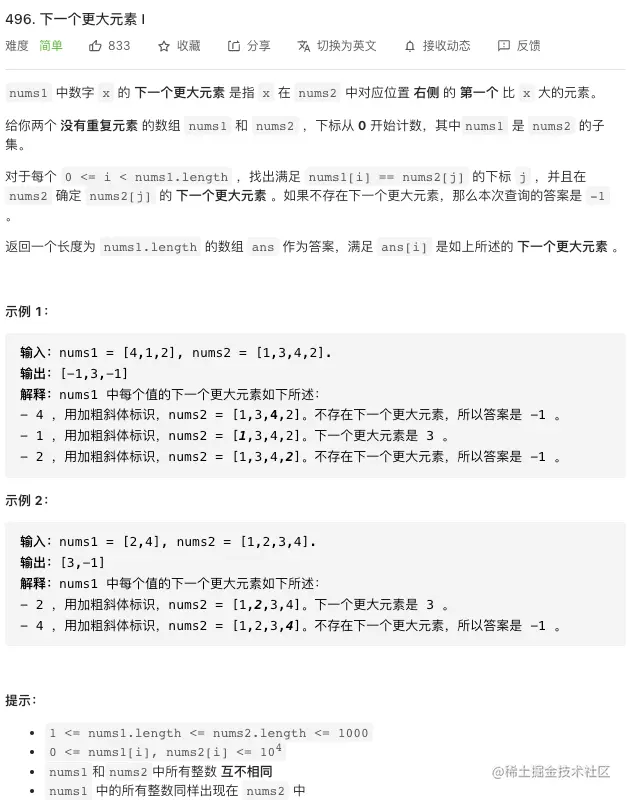• 两个没有重复元素的数组 `nums1`和 `nums2`
• `nums1``nums2` 的子集。

`题解`

``````class Solution {
fun nextGreaterElement(nums1: IntArray, nums2: IntArray): IntArray {
// 临时记录
val map = HashMap<Int, Int>()
// 单调栈
val stack = ArrayDeque<Int>()
// 从前往后查询
for (index in 0 until nums2.size) {
// while：当前元素比栈顶元素大，说明找到下一个更大元素
while (!stack.isEmpty() && nums2[index] > stack.peek()) {
// 输出到临时记录中
map[stack.pop()] = nums2[index]
}
// 当前元素入队
stack.push(nums2[index])
}
return IntArray(nums1.size) {
map[nums1[it]] ?: -1
}
}
}``````

# 6. 典型例题 · 下一个更大元素 II（环形数组）

`LeetCode 例题`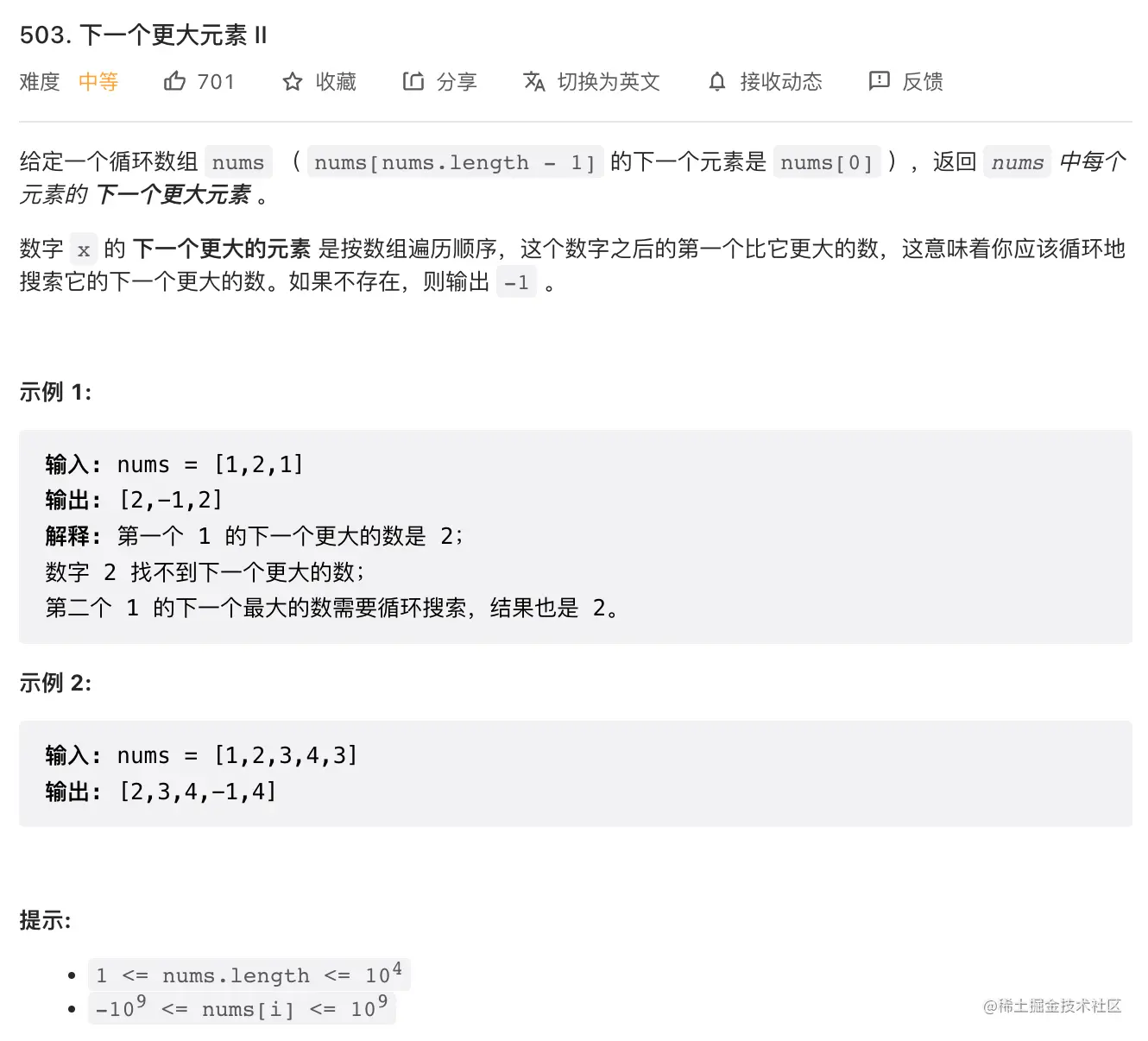`无限轮播伪代码`

``````class LooperView {
private val data = listOf("1", "2", "3")
// 假数据长度
fun getSize() = Integer.MAX_VALUE
// 使用取余转化为 data 上的下标
fun getItem(index : Int) = data[index % data.size]
}``````

`题解`

``````class Solution {
fun nextGreaterElements(nums: IntArray): IntArray {
// 结果数组
val result = IntArray(nums.size) { -1 }
// 单调栈
val stack = ArrayDeque<Int>()
// 数组长度
val size = nums.size
// 从前往后遍历
for (index in 0 until nums.size * 2) {
// while：当前元素比栈顶元素大，说明找到下一个更大元素
while (!stack.isEmpty() && nums[index % size] > nums[stack.peek() % size]) {
result[stack.pop() % size] = nums[index % size]
}
// 当前元素入队
stack.push(index)
}
return result
}
}``````

# 7. 总结

2 年后重新思考，我不再持有此观点。我现在认为：单调栈的关键是 “单调性”，而栈只是为了配合问题对操作顺序的要求而搭配的数据结构。 我们学习单调栈，应该当作学习单调性的思想在栈这种数据结构上的应用，而不是学习一种新的数据结构。对此，你怎么看？

496. 下一个更大元素 IEasy【题解】
1475. 商品折扣后的最终价格Easy【题解】
503. 下一个更大元素 IIMedium【题解】
739. 每日温度Medium【题解】
901. 股票价格跨度Medium【题解】
1019. 链表中的下一个更大节点Medium【题解】
402. 移掉 K 位数字Medium【题解】
42. 接雨水Hard【题解】
84. 柱状图中最大的矩形Hard【题解】